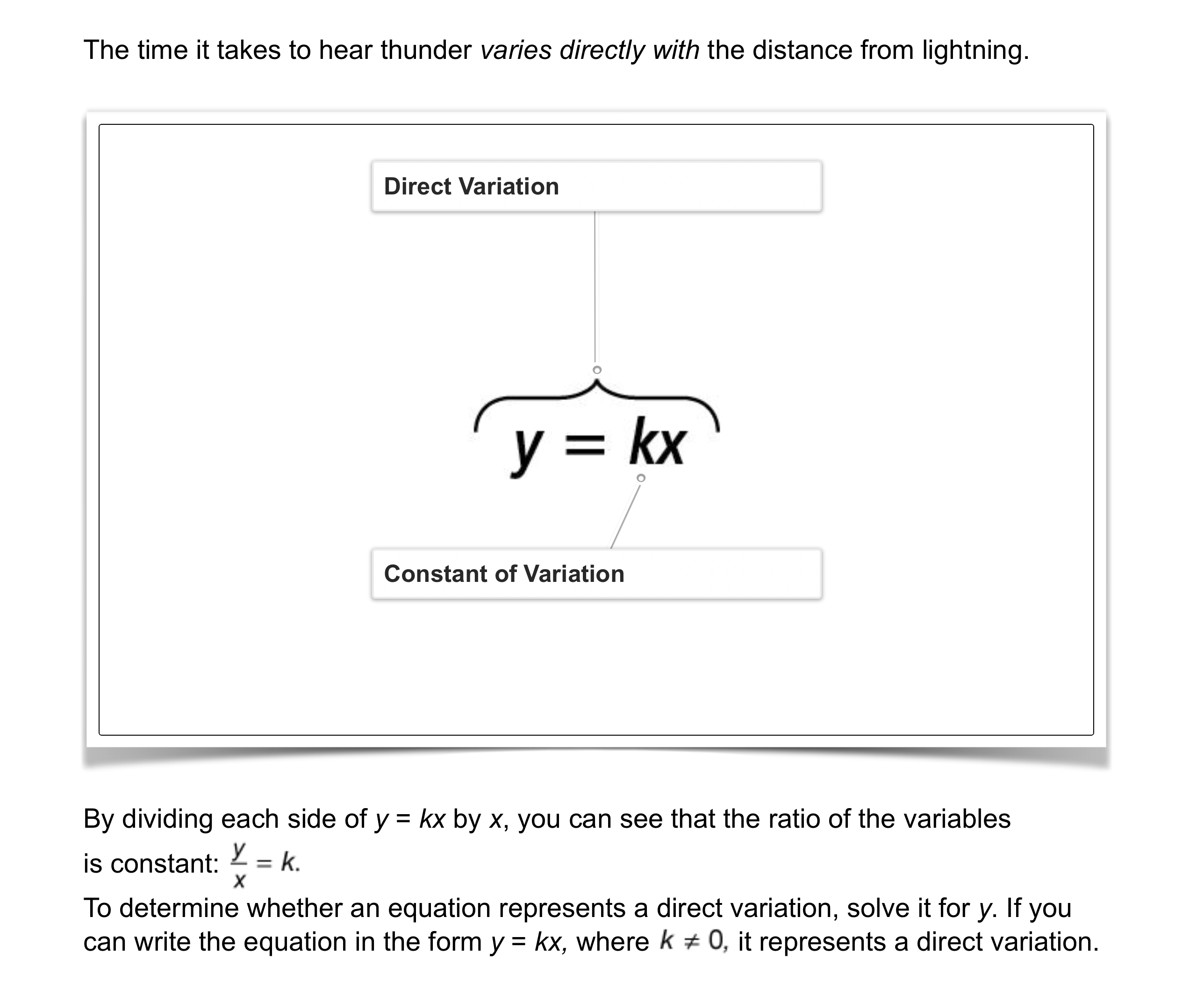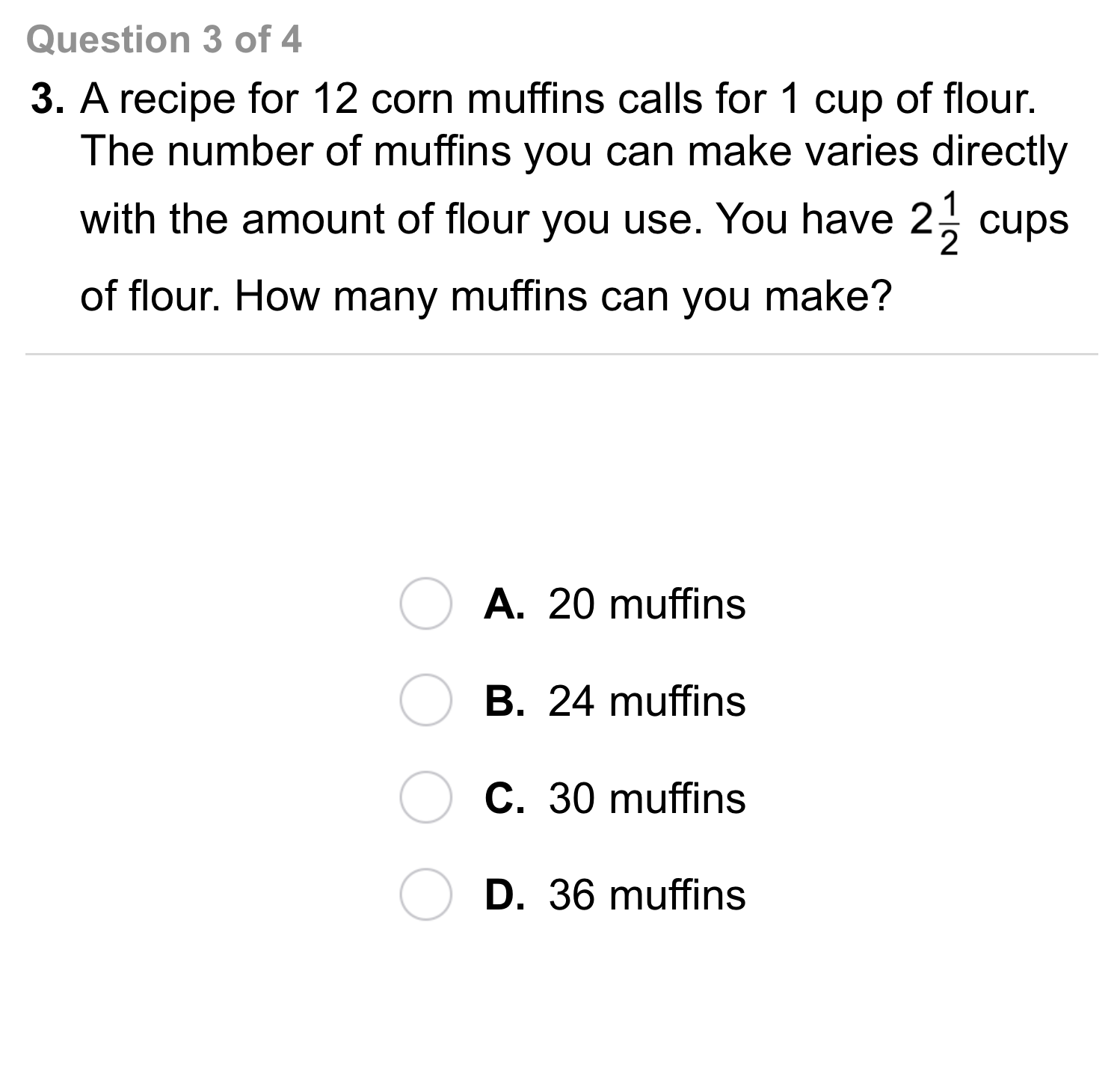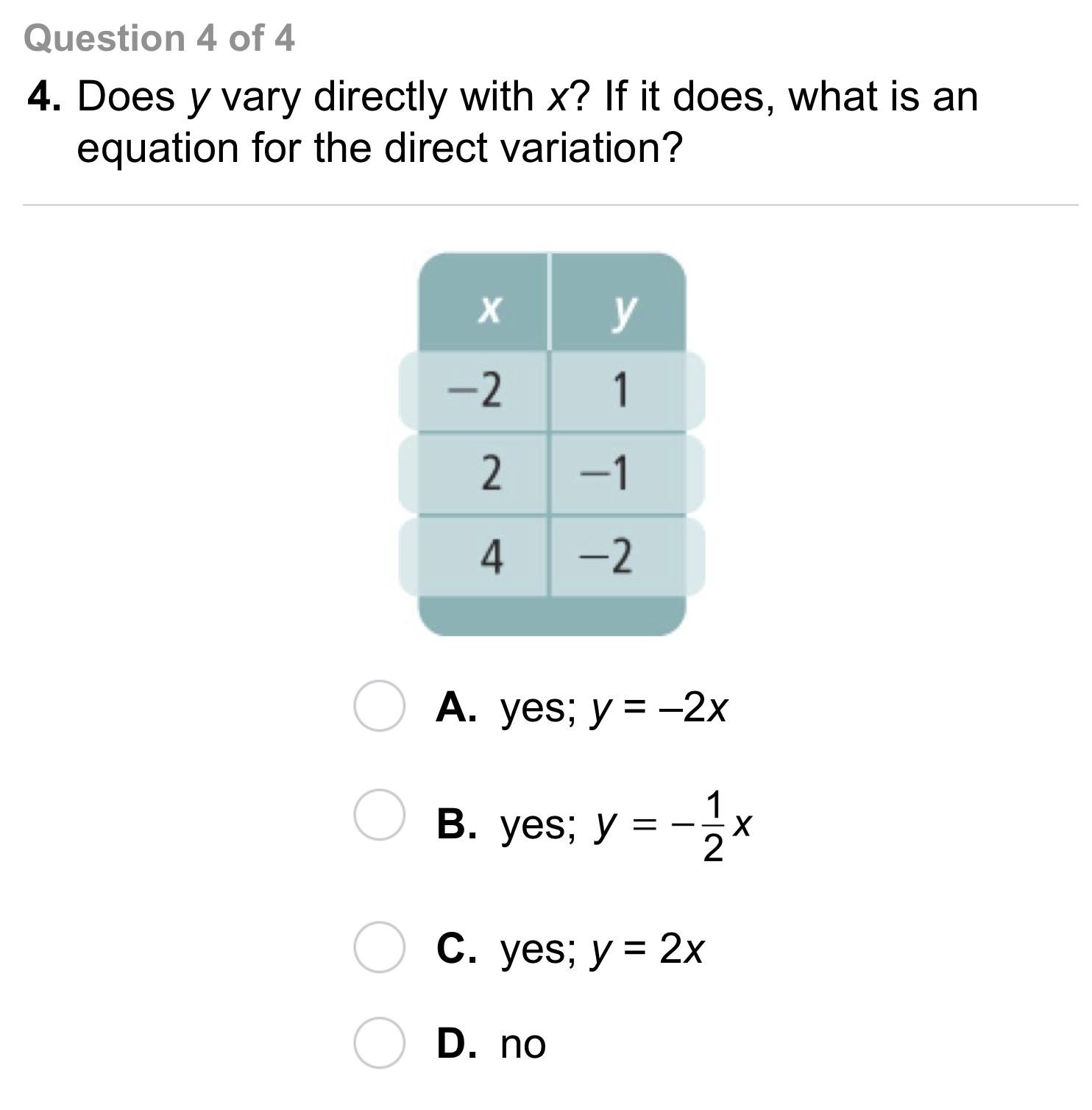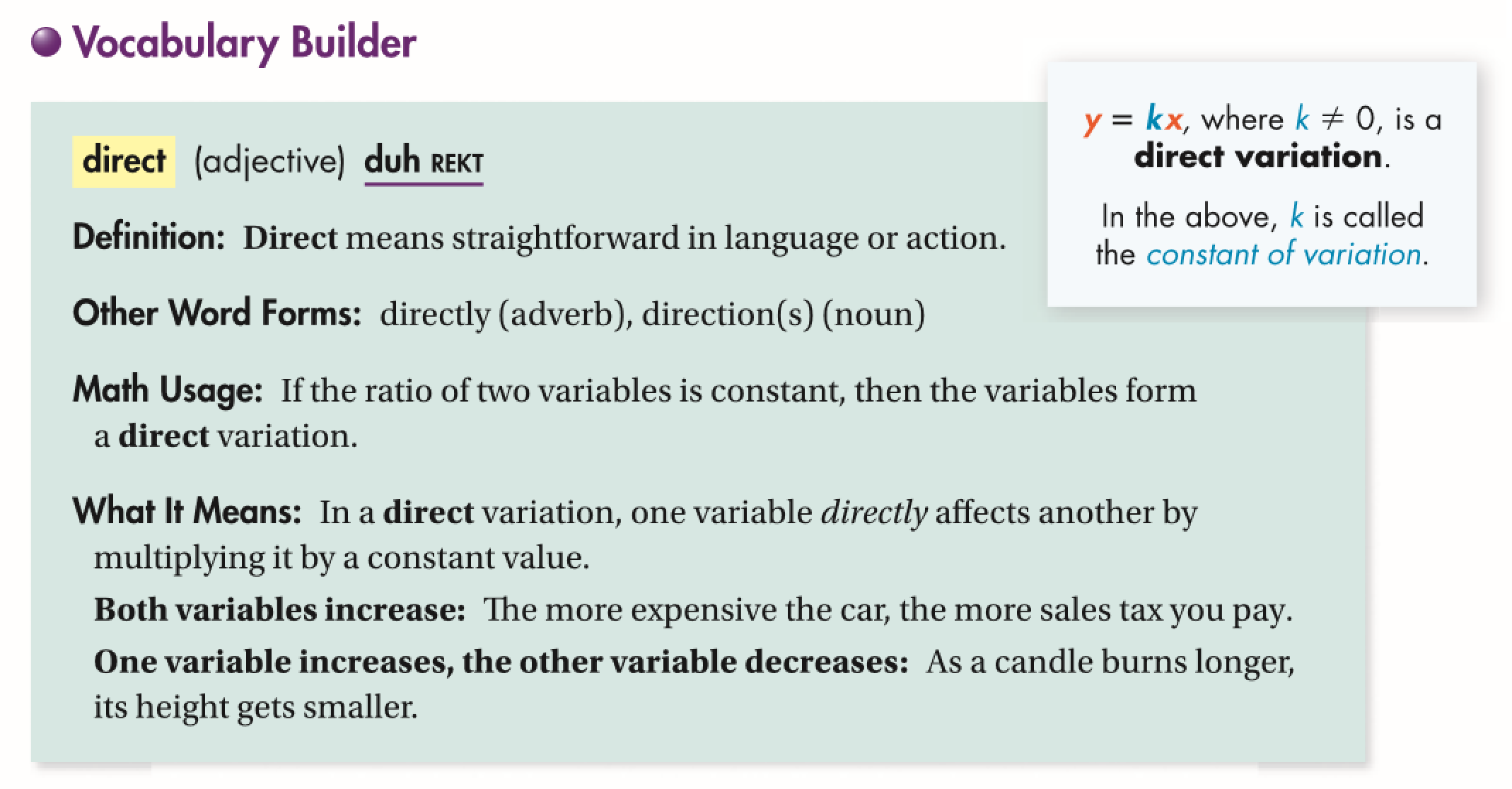Algebra 1 5-2 Complete Lesson: Direct Variation
starstarstarstarstarstarstarstarstarstar
5 (1 rating)
by Matthew Richardson
| 24 Questions
Note from the author:
A complete formative lesson with embedded slideshow, mini lecture screencasts, checks for understanding, practice items, mixed review, and reflection. I create these assignments to supplement each lesson of Pearson's Common Core Edition Algebra 1, Algebra 2, and Geometry courses. See also mathquest.net and twitter.com/mathquestEDU.The outlined content above was added from outside of Formative.1
1
10 pts
Solve It! The diagram shows how long it takes to hear thunder after you see lightning. What general rule can you use to model this situation?2
2
10 pts
Problem 1 Got It?
A
B
C
D3
3
10 pts
Problem 2 Got It?
A
B
C
D
4
20 pts
Problem 3 Got it? Weight on the moon y varies directly with weight on Earth x. A person who weighs 100 lb on Earth weighs 16.6 lb on the moon. What is an equation that relates weight on earth x and weight on the moon y? What is the graph of this equation?

Be sure to include relevant graph detail: label axes, indicate units on both axes, and use arrows to represent end behavior, as appropriate.5
5
10 pts
Problem 4 Got It?
A
B
C
D6
6
10 pts
A
B
C
D7
7
10 pts
A
B
C
D8
8
10 pts
A
B
C
D9
9
10 pts
A
B
C
D10
5 pts
Vocabulary: Determine whether each statement is always, sometimes, or never true.

The ordered pair (0, 0) is a solution of the direct variation equation y = kx.
Always
Sometimes
Never
11
5 pts
Vocabulary: Determine whether each statement is always, sometimes, or never true.

You can write a direct variation in the form below.

Always
Sometimes
Never
12
5 pts
Vocabulary: Determine whether each statement is always, sometimes, or never true.

The constant of variation for a direct variation represented by y = kx is y/x.

Always
Sometimes
Never
13
10 pts
Reasoning: Suppose q varies directly with p. Does this imply that p varies directly with q? Explain.14
10 pts
Review Lesson 5-1: Find the slope of the line that passes through the pair of points.

2
-2
-1
1
15
10 pts
Review Lesson 5-1: Find the slope of the line that passes through the pair of points.
Enter only a number. If necessary, use fraction form.
16
10 pts
Review Lesson 5-1: Find the slope of the line that passes through the pair of points.
Enter only a number. If necessary, use fraction form.
17
10 pts
Review Lesson 1-2: Evaluate the expression for the given value of the variable.

6a + 3 for a = 2
9
65
15
12
18
10 pts
Review Lesson 1-2: Evaluate the expression for the given value of the variable.

-2x - 5 for x = 3

Enter only a number.
19
10 pts
Review Lesson 1-2: Evaluate the expression for the given value of the variable.

¼x + 2 for x = 16

Enter only a number.
20
10 pts
Review Lesson 1-2: Evaluate the expression for the given value of the variable.

8 - 5n for n = 3

Enter only a number.21
10 pts
Vocabulary Review: Classify each formula from the image below as representing slope or not representing slope.

• Formula A
• Formula B
• Formula C
• Represents slope
• Does NOT represent slope22
10 pts
Use Your Vocabulary: Drag the correct word from the list to complete each sentence.
• directly
• direct
• directions
• Renee gave the visitor __?__ to the museum.
• The fans went __?__ to their seats.
• There is a __?__ connection between the outside temperature and the number of people at the beach.
23
100 pts
Notes: Take a clear picture or screenshot of your Cornell notes for this lesson. Upload it to the canvas. Zoom and pan as needed.